# Python tkinter grid

Tkinter Tutorials

Layout of the page to place the components in rows and columns. We will keep one Label along with an Entry box.

## Understanding row and column

Tkinter Grid layout management by row & columns & aligning in both horizontal & vertical directions

Horizontal placement is done by rows and vertical placement is done by columns.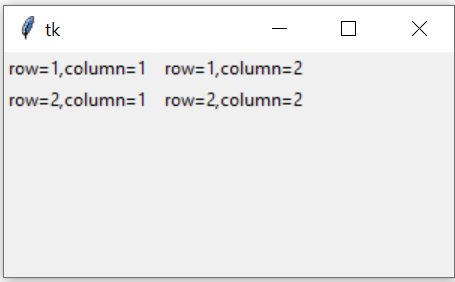``````import tkinter as tk
my_w = tk.Tk()
my_w.geometry("300x200")

l1 = tk.Label(my_w, text='row=1,column=1 ')
l1.grid(row=1,column=1)

l2 = tk.Label(my_w, text=' row=1,column=2')
l2.grid(row=1,column=2)

l3 = tk.Label(my_w, text='row=2,column=1 ')
l3.grid(row=2,column=1)

l4 = tk.Label(my_w, text=' row=2,column=2')
l4.grid(row=2,column=2)

my_w.mainloop()``````
The top line is row=1 and second line is row=2, similarly first vertical column is column=1 and next vertical column is column=2

## sticky option

If the component size is less than the available grid space, then we can use 'E','W','N','S' to align right left top and bottom.

Check F Name and L Name is aligned to left and right. We can align the components to top and bottom also.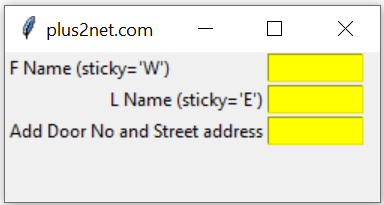``````import tkinter as tk
my_w = tk.Tk()
my_w.geometry("250x100")
my_w.title("plus2net.com")  # Adding a title

l1 = tk.Label(my_w,  text='F Name (sticky=\'W\')' ) #added one Label
l1.grid(row=1,column=1,sticky='W')

e1 = tk.Entry(my_w,   width=10,bg='yellow') # added one Entry box
e1.grid(row=1,column=2)

l2 = tk.Label(my_w,  text='L Name (sticky=\'E\')')  #
l2.grid(row=2,column=1,sticky='E')

e2 = tk.Entry(my_w,   width=10,bg='yellow') # added one Entry box
e2.grid(row=2,column=2)

l3 = tk.Label(my_w,  text='Add Door No and Street address')
l3.grid(row=3,column=1,sticky='W')

e3 = tk.Entry(my_w,   width=10,bg='yellow') # added one Entry box
e3.grid(row=3,column=2)

my_w.mainloop()``````

## columnspan option

Tkinter window grid layout using rowspan and columnspan to manage multiple rows and columns

By default `columnspan=1`, we can set to different value by increasing it to expand more than one column.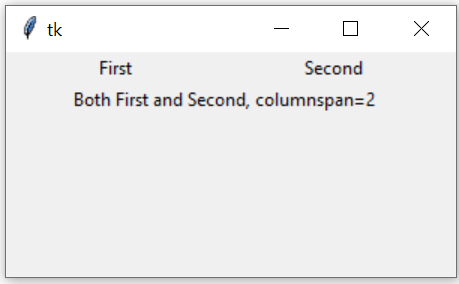``````l1 = tk.Label(my_w, text='First', width=20)
l1.grid(row=1,column=1)
l2 = tk.Label(my_w, text='Second', width=20)
l2.grid(row=1,column=2)

l3 = tk.Label(my_w, text='Both First and Second, columnspan=2')
l3.grid(row=2,column=1,columnspan=2)``````

## rowspan options

We can use `rowspan=3` to expand to three rows.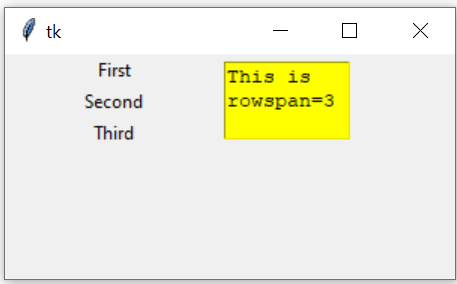``````import tkinter as tk
my_w = tk.Tk()
my_w.geometry("300x150")
l1 = tk.Label(my_w, text='First', width=20)
l1.grid(row=1,column=1)
l2 = tk.Label(my_w, text='Second', width=20)
l2.grid(row=2,column=1)
l3 = tk.Label(my_w, text='Third', width=20)
l3.grid(row=3,column=1)

t1 = tk.Text(my_w,  height=3, width=8,bg='yellow') #text box
t1.grid(row=1,column=2,rowspan=3)

my_w.mainloop()``````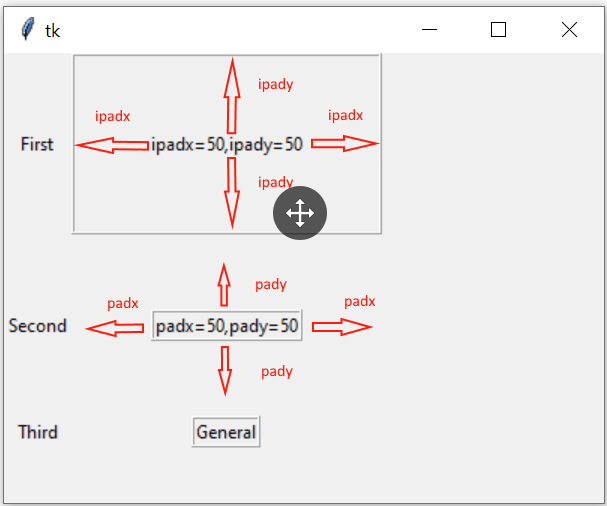``````l4=tk.Label(my_w,text='ipadx=50,ipady=50',
borderwidth=2,relief='ridge')
``````l4=tk.Label(my_w,text='padx=50,pady=50',
borderwidth=2,relief='ridge')

## grid_size()

This will return a tuple showing first empty columns and rows . In this example we have used upto row=3 and column=3 so we will get output as (4,4).
``````import tkinter as tk
my_w = tk.Tk()
my_w.geometry("300x150")
l1 = tk.Label(my_w, text='First', width=8)
l1.grid(row=1,column=1)
l2 = tk.Label(my_w, text='Second', width=8)
l2.grid(row=2,column=2)
l3 = tk.Label(my_w, text='Third', width=8)
l3.grid(row=3,column=3)

print(my_w.grid_size()) # tuple  columns , rows

my_w.mainloop()``````
Output
``(4, 4)``
You can remove the last element and check the output. We will set the value as `column=2` for last element.
``````l3 = tk.Label(my_w, text='Third', width=8)
l3.grid(row=3,column=2) ``````
Output
``(3, 4)``

## grid_slaves(row=None, column=None)

We will get a list of widgets manage by the grid.
``````import tkinter as tk
my_w = tk.Tk()
my_w.geometry("300x150")
l1 = tk.Label(my_w, text='First', width=8)
l1.grid(row=1,column=1)
l2 = tk.Label(my_w, text='Second', width=8)
l2.grid(row=2,column=2)
l3 = tk.Label(my_w, text='Third', width=8)
l3.grid(row=3,column=2)

print(my_w.grid_slaves())

my_w.mainloop()``````
Output
``````[<tkinter.Label object .!label3>, <tkinter.Label object .!label2>,
<tkinter.Label object .!label>]``````
We can use row and column number to get the widget.
``print(my_w.grid_slaves(2,2)) ``
Output
``[<tkinter.Label object .!label2>]``

## grid_remove()

Remove the widget from the perticular grid. Can be added again.
``````import tkinter as tk
my_w = tk.Tk()
my_w.geometry("300x150")
l1 = tk.Label(my_w, text='First', width=8)
l1.grid(row=1,column=1)
l2 = tk.Label(my_w, text='Second', width=8)
l2.grid(row=2,column=2)
l3 = tk.Label(my_w, text='Third', width=8)
l3.grid(row=3,column=2)
l3.grid_remove()
my_w.mainloop()``````
To restore the widget in same location we have to just use grid()
``l3.grid()``

## grid_forget()

Remove the widget from the perticular grid. Can be added again at the new location. We have to specify the location while restoring, by default it takes the next new location.
``l3.grid_forget()``
To restore at the same location we have to specify the row and column.
``l3.grid(row=3,column=2)``

## Removing after some time delay

We can use the widget as message box and want to remove it after 300 seconds.
``l3.after(3000, lambda: l3.grid_remove() )``

## Remove all widgets

``````for w in my_w.grid_slaves(): # Loop through each row
w.grid_forget() # remove the row``````

## Remove all widgets of 2nd row only

``````for w in my_w.grid_slaves(2):
w.grid_forget() ``````

## Remove all widgets of 2nd column

``````for w in my_w.grid_slaves(None,2):
w.grid_forget()``````

## Delete widgets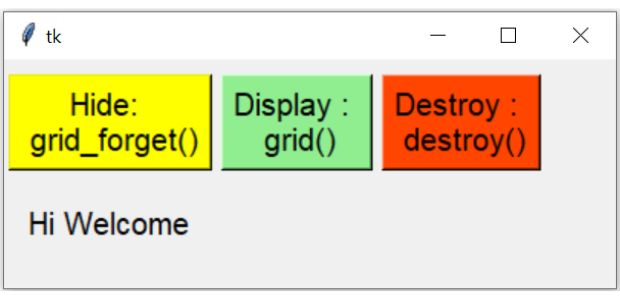By using destroy() method we can delete a widget permanently. We can't restore it again.
``````import tkinter as tk
my_w = tk.Tk()
my_w.geometry("300x150")

b1=tk.Button(my_w,text='Hide: \n grid_forget()',
command=lambda:l1.grid_forget())
b2=tk.Button(my_w,text='Display : \n grid()',
command=lambda:l1.grid())
b3=tk.Button(my_w,text='Destroy : \n destroy()',
command=lambda:l1.destroy())

l1 = tk.Label(my_w, text='Hi Welcome', width=10)
my_w.mainloop()``````

## grid_info()

All information about the widget layout in the grid.
``````import tkinter  as tk
from tkinter import *
my_w = tk.Tk()
my_w.geometry("400x200")  # Size of the window
my_w.title("www.plus2net.com")  # Adding a title
l1 = tk.Label(my_w, text='row=1,column=1 ')

print(l1.grid_info()) # dictionary all elements value
print(l1.grid_info()['column'])# 1

my_w.mainloop()  # Keep the window open``````

Subscribe to our YouTube Channel here

## Subscribe

* indicates required
Subscribe to plus2netplus2net.com

Post your comments , suggestion , error , requirements etc here